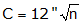## 2. Angular Misclosure

Angular misclosure is the difference between the measured angles' sum on a traverse and the angle condition for the traverse configuration.
The amount of allowable angular misclosure is dependent on the survey purpose. The limits are generally expressed as an Error of a Series:Equation B-4 C: Allowable misclosure, seconds k: expected error in each angle, seconds n: number of angles

Traditional formal standards from the FGCS Standards and Specifications for Geodetic Control Networks are:

 Table B-1 Angular Misclosure Order Class k (sec) First - 1.7 Second I 3.0 II 4.5 Third I 10.0 II 12.0

Example

What is the allowable angular misclosure for a five-sided traverse if it is to meet Second Order Class II standards, Figure B-5?Figure B-5 Allowable Misclosure

From Table 1, k = 4.5". Substituting into Equation B-4:Because this is a five-sided loop traverse, using Equation B-1 the angles should sum to:Therefore, the total should be in the range 539°59'50" to 540°00'10".

Informal standards can be created which reflect equipment, experience, conditions, project needs, etc.

Example

A control network is to be established for a construction project. Sight distances will be relatively short (under 300 ft) so measuring angles consistently will be a challenge. Testing under these conditions, our survey crew was able to measure an angle to a consistency of ±0°00'12". For this project, we will use an allowable misclosure of: